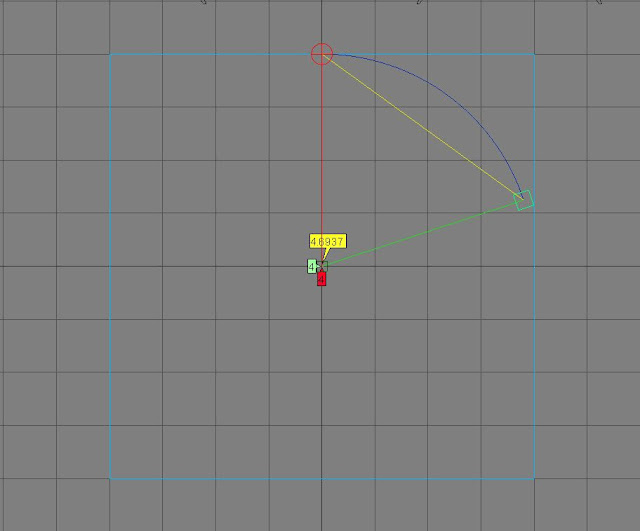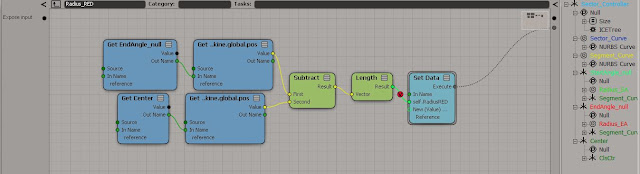## Tuesday, April 9, 2013

### Sector/Segment Area

There are two main "slices" of a circle:
The "pizza" slice is called a Sector*white selection below**Click to make bigger*

And the slice made by a chord is called a Segment. *white selection below**Click to make bigger*

Lets take a look at this in Softimage....

* Watch in highest quility possible*

To work out the area of the segment and sector you will use the formulas:

Area of Sector = ½ × (θ × π/180) × r2   (when θ is in degrees)

Area of Segment = ½ × ( (θ × π/180) - sin θ) × r2   (when θ is in degrees)

*So basically what we need is the angle of the "center" corner, and the radius of the circle the sector belongs too**Click to make bigger*

What these formulas will look like in ICE - once you have the angle and raduis - :

Segment area:*Click to make bigger*

Sector area:*Click to make bigger*

So you will need to work out the lengths of the three sides of the triangle that fits inside the sector "pizza slice".
I did this by simple creating cluster centers on the last point of the three curves then getting the length between them....*Click to make bigger*

Here are the tree's for that...*Click to make bigger*
they are all basically the same...*Click to make bigger**Click to make bigger*

....then you can work out the angle of the center corner in the circle your sector is part of.
I used the standard law of cosines node for this one but here is the math formula:
cos A = (b2 + c2 - a2)/2bc

I used this law because I already had all three side lengths worked out, but never fear here is a link to other ways of working out you triangles if you have say one angle and two sides...*Click to make bigger**Click to make bigger*

You can also work out the "arc length" - the blue line in the image below - of your sector, by using the formula:

L = (θ × π/180) × r*Click to make bigger*

Here's that ICE tree...*Click to make bigger*

And there you have it. If you ever need to work out how big a pizza slice is ..... now you can do it :)

End